Test: Electrostatics- 2

# Test: Electrostatics- 2

Test Description

## 20 Questions MCQ Test Topicwise Question Bank for Electrical Engineering | Test: Electrostatics- 2

Test: Electrostatics- 2 for Electrical Engineering (EE) 2023 is part of Topicwise Question Bank for Electrical Engineering preparation. The Test: Electrostatics- 2 questions and answers have been prepared according to the Electrical Engineering (EE) exam syllabus.The Test: Electrostatics- 2 MCQs are made for Electrical Engineering (EE) 2023 Exam. Find important definitions, questions, notes, meanings, examples, exercises, MCQs and online tests for Test: Electrostatics- 2 below.
Solutions of Test: Electrostatics- 2 questions in English are available as part of our Topicwise Question Bank for Electrical Engineering for Electrical Engineering (EE) & Test: Electrostatics- 2 solutions in Hindi for Topicwise Question Bank for Electrical Engineering course. Download more important topics, notes, lectures and mock test series for Electrical Engineering (EE) Exam by signing up for free. Attempt Test: Electrostatics- 2 | 20 questions in 60 minutes | Mock test for Electrical Engineering (EE) preparation | Free important questions MCQ to study Topicwise Question Bank for Electrical Engineering for Electrical Engineering (EE) Exam | Download free PDF with solutions
 1 Crore+ students have signed up on EduRev. Have you?
Test: Electrostatics- 2 - Question 1

### Which one of the following pairs is NOT correctly matched?

Detailed Solution for Test: Electrostatics- 2 - Question 1

Option (c) is Faraday's law given by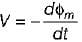Test: Electrostatics- 2 - Question 2

### Match List-I (Law/Quantity) with List-ll (Mathematical expression) and select the correct answer using the codes given below the lists: List-I A. Gauss’s law B. Ampere’s law C. Faraday’s law D. Poynting vector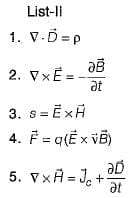Codes:     A B C D (a) 1 2 4 3 (b) 3 5 2 1 (c) 1 5 2 3 (d) 3 2 4 1

Test: Electrostatics- 2 - Question 3

### What is the value of total electric flux coming out of a closed surface?

Detailed Solution for Test: Electrostatics- 2 - Question 3

From Gauss’s law, total electric flux from a closed surface is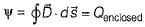.

Test: Electrostatics- 2 - Question 4

Which one of the following is the Poisson's equation for a linear and isotropic but in homogeneous medium?

Detailed Solution for Test: Electrostatics- 2 - Question 4

For homogeneous medium ∇2 V = -ρ∈ and for non-homogeneous medium ∈ is a variable quantity due to which Poisson’s equation becomes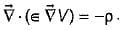Test: Electrostatics- 2 - Question 5

Two concentric spherical shells carry equal and opposite uniformly distributed charges over their surfaces as shown in figure below.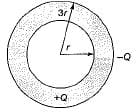Electric field on the surface of inner shell will be

Test: Electrostatics- 2 - Question 6

Electric field intensity due to line charge of infinite length is

Test: Electrostatics- 2 - Question 7

Consider the following statements associated with equipotential surface:
1. Potential is same everywhere.
2. No current flows on this surface.
3. Work done in moving charge from one point to another is zero.
4. Potential is different everywhere.
Which of the above statements is/are not correct?

Detailed Solution for Test: Electrostatics- 2 - Question 7

Potential is same everywhere on an equipotential surface. Hence, statement 4 is not correct. Statements 1,2 and 3 are correct.

Test: Electrostatics- 2 - Question 8

A finite sheet 0 ≤ x ≤ 1, 0 ≤ 1 on the z = 0 plane has a charge density
ρs = xy (x2 + y2 + 25)3/2 nC/m2.
The total charge on the sheet would be

Detailed Solution for Test: Electrostatics- 2 - Question 8

The total charge on the sheet is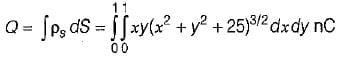Since,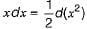, we now integrate w.r.t. x
(or change variables: x2 = u so that xdx = du/2)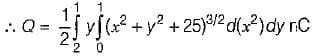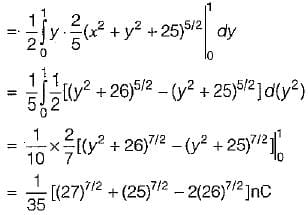Test: Electrostatics- 2 - Question 9

Assertion (A): Gauss’s law is an alternative statement of Coulomb’s law.
Reason (R): Proper application of the divergence theorem to Coulomb’s law results in Gauss’s law.

Test: Electrostatics- 2 - Question 10

If the potential V = 10/r2 sinθcosϕ then the work done in moving a 10 μC charge from point A(1, 30°, 120°) to B(4, 90°, 60°) would be equal to

Detailed Solution for Test: Electrostatics- 2 - Question 10

Required work done,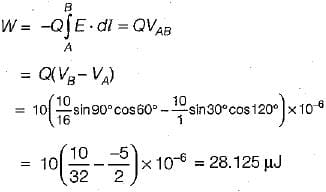Test: Electrostatics- 2 - Question 11

A wire of diameter 1 mm and conductivity 5 x 107S/m has 1029 free electrons /mwhen an electric field of 10 mV/m is applied.
The current density and the drift velocity of the electrons will be respectively given by (take charge on an electrons e = -1.6 x 10-19 C)

Detailed Solution for Test: Electrostatics- 2 - Question 11

The charge density of free electron is:
ρv = ne = (1029) x (-1.6 x 10-19)
= -1.6 x 1010 C/m2
Given,
E = 10 mV/m,
d = 1 mm,
σ = 5 x 107 S/m,
n = 1029/m3
Now, current density,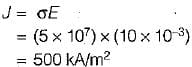Also, drift velocity,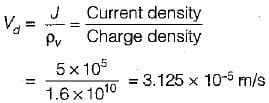Test: Electrostatics- 2 - Question 12

The equivalent capacitance between the terminals A and B for the capacitor shown below would be (the area occupied by each dielectric is 30 cm2)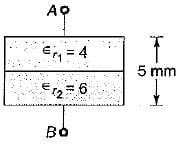Detailed Solution for Test: Electrostatics- 2 - Question 12

Given,
d = 5 mm, d/2 = 2.5 mm,
f1 = 4, ∈f2 = 6,
A/2 = 15 cm2
For the given arrangement of dielectrics, D and E are parallel to the dielectric interface. Therefore, we can treat the capacitor as consisting of two capacitors C1 and C2 in parallel (same voltage across C1 and C2).
Thus,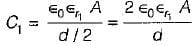and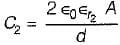The total capacitance/equivalent capacitance between terminals A and B is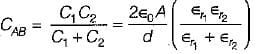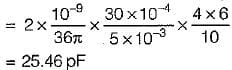Test: Electrostatics- 2 - Question 13

Three point charges -1 nC, 4 nC and 3 nC are located at (0, 0, 0) , (0,0,1) and (1,0,0,) respectively. To total energy contained in the system would be

Detailed Solution for Test: Electrostatics- 2 - Question 13

Total energy contained in the system is:
W=W+ W+ W3
= 0 + Q2V21 + Q3 (V31 + V32)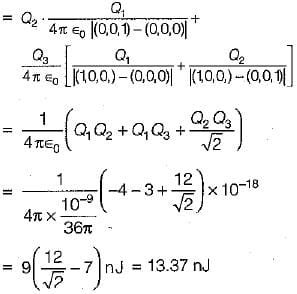Test: Electrostatics- 2 - Question 14

The cylindrical coordinates equation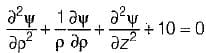is called

Test: Electrostatics- 2 - Question 15

Image theory is applicable to problems involving

Test: Electrostatics- 2 - Question 16

Match List-I with List-ll and select the correct answer using the codes given below the lists:
List-I
A. Joule’s law
B. Ohm’s law
C. Solenoidal current
D. Continuity equation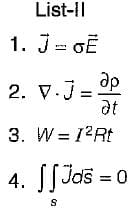Codes:
A B C D
(a) 4 2 3 1
(b) 3 1 2 4
(c) 3 1 4 2
(d) 4 3 1 2

Test: Electrostatics- 2 - Question 17

Assertion (A): An electric field produces no migration of charge in a dieiectric.
Reason (R): The permittivity of a dielectric is always less than the permittivity of vaccum.

Detailed Solution for Test: Electrostatics- 2 - Question 17
• Assertion is a true statement.
• Reason is a false statement because the permittivity of dielectric is always more than the permittivity of vaccum which makes it a good insulator.
• In a dielectric or insulator the electrons are so tightly bound by their parent nuclei in the equilibrium positions that they can not easily be detached by the applications of electric field. Due to this reason an electric field produces no migration of charge in a dielectric.
Test: Electrostatics- 2 - Question 18

The potential field at any point in space containing a dielectric material of relative permittivity 3 is given by V = (5x2y + 3yz2 + 6xz) Volts, where x, y, z are in meters. The magnitude of volume charge density at point (1,2, 3) will be given by

Detailed Solution for Test: Electrostatics- 2 - Question 18

Given, V = (5x2y + 3yz2 + 6xz) Volts and
r = 3
Using Poisson’s equation,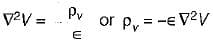Here,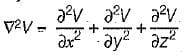= 10y + 0 + 6y = 16y
So,  ρv = -∈ (10y+6y)
∴  ρv|at (1,2,3) = -∈0r (10 x 2 + 6 x 2)
= -∈0 x 3 x 32 = - 96∈0
= 96∈0 (Magnitude only)

Test: Electrostatics- 2 - Question 19

If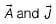are the vector potential and current density vectors associated with a coil, then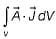has the unit of

Detailed Solution for Test: Electrostatics- 2 - Question 19

On comparing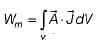with electrical energy stored i.e.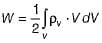we can find that Wm has the unit of energy.

Test: Electrostatics- 2 - Question 20

In the infinite plane, y = 6 m, there exists a uniform surface charge density of (1/600π) μC/m2 . The associated electric field strength is

Detailed Solution for Test: Electrostatics- 2 - Question 20

Given, y = 6 cm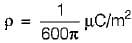The electric field strength due to an infinite charged sheet is given by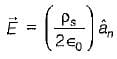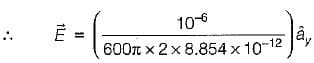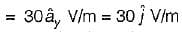## Topicwise Question Bank for Electrical Engineering

207 tests
 Use Code STAYHOME200 and get INR 200 additional OFF Use Coupon Code
Information about Test: Electrostatics- 2 Page
In this test you can find the Exam questions for Test: Electrostatics- 2 solved & explained in the simplest way possible. Besides giving Questions and answers for Test: Electrostatics- 2, EduRev gives you an ample number of Online tests for practice

207 tests